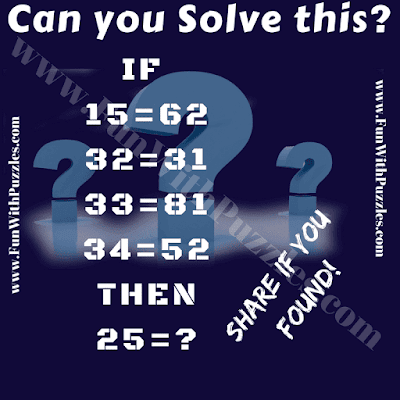Here is Brain teasing Maths Question for school going teens. In this mind teasing Maths Question, you are given simple number equations. However numbers on both sides of these equations has some logical relationship with each other. Your challenge is to decipher this logical sequence or pattern in these equations are then find the value of missing number which is answer of last equation. Lets see how much your will take to solve this Brain Teasing Maths Question?Can you solve this brain teasing Maths Question?

Answer of this "Brain Teasing Maths Question", can be viewed by clicking on button. Please do give your best try before looking at the answer.

Unknown said...

False